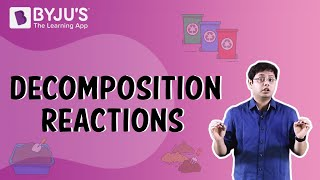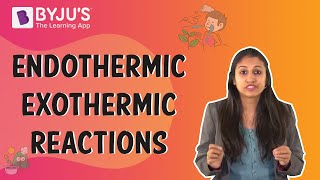# Decomposition Reaction Questions

Atoms of one element do not transform into those of another during a chemical reaction. Atoms do not vanish from the mixture or arrive from somewhere else. In chemical reactions, in fact, bonds between atoms are broken and formed during reactions generating new substances.

 Definition: When a single reactant breaks down to give simpler products then the reaction is said to be a decomposition reaction.

## Decomposition Reaction Chemistry Questions with Solutions

Q-1: Decomposition reactions are generally

1. Exothermic
2. Oxidative
3. Reductive
4. Endothermic

Explanation:

Energy is required to break bonds, whereas energy is released when bonds are formed. A decomposition process requires breaking the bonds of a big molecule to generate two or more smaller molecules, making the reaction endothermic.

Q-2: Which of the following is produced upon thermal decomposition of zinc sulphate?

1. Zinc oxide
2. Sulphur trioxide
3. Both of the above
4. Sulphur dioxide

Answer: c) Both of the above

Explanation: Zinc sulphate undergoes thermal decomposition in the following way:

$$\begin{array}{l}ZnSO_{4}\overset{\Delta }{\rightarrow}ZnO + SO_{3}\end{array}$$

Q-3: What are the three different types of decomposition reactions? State one example for each.

Answer: The three types of decomposition reactions are:

1. Thermal Decomposition Reaction: A thermal decomposition reaction necessitates the delivery of heat energy to the reactants. When calcium carbonate is heated, it decomposes into calcium oxide and carbon dioxide, which is a common example of a thermal decomposition reaction.
2. Photolytic Decomposition Reaction: A photodecomposition reaction is a sort of decomposition reaction in which the reactant absorbs energy from photons and breaks down into its parts. Decomposition of ozone into dioxygen and an oxygen radical is an example of a photo decomposition reaction.
3. Electrolytic Decomposition Reaction:The activation energy for decomposition is delivered in the form of electrical energy in an electrolytic decomposition reaction. The electrolysis of water is one type of electrolytic breakdown reaction.

Q-4: Which reaction is the opposite of the decomposition reaction?

1. Displacement reaction
2. Disproportionation Reaction
3. Combination Reaction
4. Precipitation Reaction

Explanation: A combination reaction involves the formation of a single product from two or more reactants.

The general reaction for such reactions is:

A+B→ AB

Q-5: State a reaction that is involved in black and white photography.

The reaction that is most commonly employed in black and white photography is:

$$\begin{array}{l}2AgCl(s)\overset{sunlight}{\rightarrow}2Ag(s)+Cl_{2}(g)\end{array}$$

Q-6: Chemical decomposition causes the compound to separate

a) physically

b) chemically

c) electrically

d) thermally

Explanation: By breaking bonds, a single molecule is degraded into two or more products in these reactions. Because chemical bonds are breaking and new ones are forming, atoms in a molecule are chemically separated.

Q-7: Which of the following conditions is not required for a decomposition reaction?

1. Only one reactant is present.
2. There are two or more products available.
3. Electricity is always required
4. All of the above conditions are required.

Answer: c) Electricity is always required.

Explanation: Electricity is not always required. Many decomposition reactions take place thermally and as well as photolytically.

Q-8: Carefully observe the activity shown below and then answer the following questions.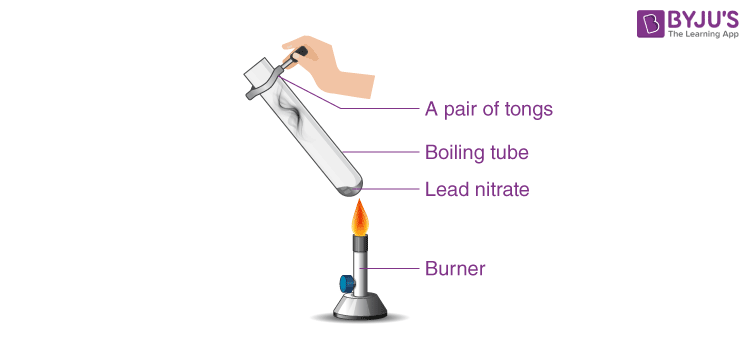1. Identify the type of the reaction
2. What do you observe? Note down the change,if any.
3. Explain the observation with the help of a chemical reaction.

1. Thermal decomposition reaction
2. The emission of brown fumes will be observed. These fumes are nitrogen dioxide (NO2 ).
3. The reaction that takes place is:

2Pb(NO3)2(s) + heat → 2PbO(s) + 4NO2(g) +O2(g)

Q-9: All decomposition reactions are redox reactions. State whether the statement is true or false.

Explanation:

A redox reaction is one in which the simultaneous reduction and oxidation process takes place.

Heating of zinc carbonate to produce zinc oxide and carbon dioxide is a decomposition reaction but not a redox reaction. There is no reduction(gain of electron) and oxidation(loss of electron) taking place.

ZnCO3→ ZnO + CO2

Taking another example of a decomposition reaction

2NaH→2Na +H2

This reaction is redox and as well as decomposition.

Hence, decomposition reactions can be redox as well but not always.

Q-10: Identify the substances reduced or oxidised in the following decomposition reactions.

1. 2KClO3 + heat → 2KCl + 3O2
2. 2KMnO4 + heat → K2MnO4 + MnO2 + O2

A specie that undergoes an increase in oxidation state is said to be oxidised.

A specie that undergoes a decrease in oxidation state is said to be reduced.

a. Oxidised specie: Oxygen atom

Reduced specie: Chlorine atomb. Oxidised specie: Oxygen atom

Reduced specie: Manganese atom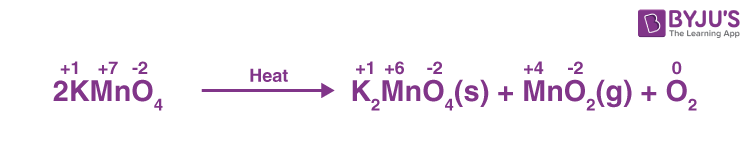Q-11: Convert the following statements into chemical equations and determine the type of chemical reaction.

1. Aluminium oxide on heating produces aluminium and oxygen gas.
2. Hydrogen sulphide gas burns in air to give water and sulphur dioxide.

Answer: Chemical equations are symbolic representations of chemical reactions that express the reactants and products in terms of their chemical formulae.

1. 2Al2O3 + heat → 4Al + 3O2 (Thermal Decomposition reaction)
2. 2H2S +3O2 → 2H2O + 2SO2 ( Redox Reaction)

Q-12: What are applications of decomposition reactions?

Answer: Decomposition reaction plays an important role in the following ways:

1. Metals are extracted from their chloride or oxide ores through electrolytic breakdown.

2. A catalytic breakdown reaction occurs when starch and other carbs are broken down into simple sugars like glucose in the presence of enzymes during digestion.

3. Photographers use decomposition reactions. The photographic film features a silver bromide layer that splits into silver and bromine when exposed to light.

Q-13: In the following decomposition reaction,

2N2O5 → 4NO2 +O2

Oxygen gas is produced at the average rate of 9.1×10-4 molL-1s-1, find the average rate of appearance of nitrogen dioxide and disappearance of nitrogen pentoxide.

Given:

$$\begin{array}{l} \frac{\Delta O_{2}}{\Delta t}\end{array}$$
= 9.1×10-4 molL-1s-1
$$\begin{array}{l}Rate=-\frac{1}{2}\frac{\Delta N_{2}O_{5}}{\Delta t} = +\frac{1}{4}\frac{\Delta NO_{2}}{\Delta t}=\frac{\Delta O_{2}}{\Delta t}\end{array}$$
Rate of the appearance of nitrogen dioxide =

$$\begin{array}{l}+\frac{1}{4}\frac{\Delta NO_{2}}{\Delta t}\end{array}$$

=9.1×10-4 molL-1s-1

$$\begin{array}{l}\frac{\Delta NO_{2}}{\Delta t}\end{array}$$

= 4×9.1×10-4

= 3.6×10-3molL-1s-1

Rate of disappearance of nitrogen pentoxide =

$$\begin{array}{l}-\frac{1}{2}\frac{\Delta N_{2}O_{5}}{\Delta t}\end{array}$$

= 9.1×10-4 molL-1s-1

$$\begin{array}{l}\frac{\Delta N_{2}O_{5}}{\Delta t}\end{array}$$

= 2×9.1×10-4

= 1.8×10-3molL-1s-1

Q-14: In which of the following decomposition reactions, the evolving gas does not have the same number of sigma and pi bonds.

1. BeCO3 + heat →
2. FeSO4 + heat→
3. (NH4)2Cr2O7 + heat →
4. ZnSO4 + heat →

Explanation:

1. BeCO3 + heat → BeO +CO2

CO2(g) has same number of sigma and pi bond equal to 2

1. FeSO4 + heat→ Fe2O3 +SO2+SO3

SO2 and SO3 have the same number sigma and pi bond equal to 2 and 3 respectively.

1. (NH4)2Cr2O7 + heat → N2 + Cr2O3 + 4H2O

N2 has one sigma and two pi bonds.

1. ZnSO4 + heat → ZnO + SO3

SO3 has the same number of sigma and pi bonds equal to 3.

Q-15: In a 500 mL evacuated vessel, two moles of ammonia are added at high temperature. 2NH3(g) ⇋ N2(g) + 3H2(g) is the decomposition reaction. NH3 becomes 1 mole at equilibrium. Determine the nitrogen and hydrogen gas equilibrium concentration.

 2NH3(g) ⇋ N2(g) + 3H2(g) Initial moles 2 – – At equilibrium 2-2x x 3x

At equilibrium, moles of ammonia =1

This implies, 2-2x =1

x= 0.5

Equilibrium moles of N2 = x= 0.5mol

Equilibrium concentration of N2 = Moles of N2/Volume( in L)

= 0.5 mol/0.5L

= 1M

Equilibrium moles of H2 = 3x= 3×0.5mol

=1.5 mol

Equilibrium concentration of H2 = Moles of H2/Volume( in L)

= 1.5 mol/0.5L

= 3M

## Practise Questions on Decomposition Reaction

Q-1: When a china dish containing 2g of silver bromide is placed in sunlight as shown in the below figure, the colour changes. What is its colour?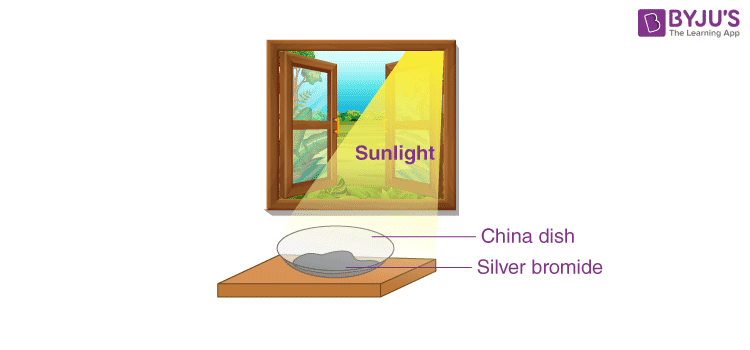Q-2: What form(s) of energy causes decomposition reaction?

1. Thermal
2. Light
3. Electrical
4. All of these

Q-3: Which reaction shows the physical decomposition?

1. CuCO3 +heat → CuO(s) + CO2 (g)
2. 2H2O + electricity → H2(g) + O2(g)
3. CuSO4.5H2O(s) + heat→ CuSO4 + 5H2O
4. 2FeSO4 + heat → Fe2O3 + SO2 + SO3

Q-4: Based on the experiment shown, answer the following questions?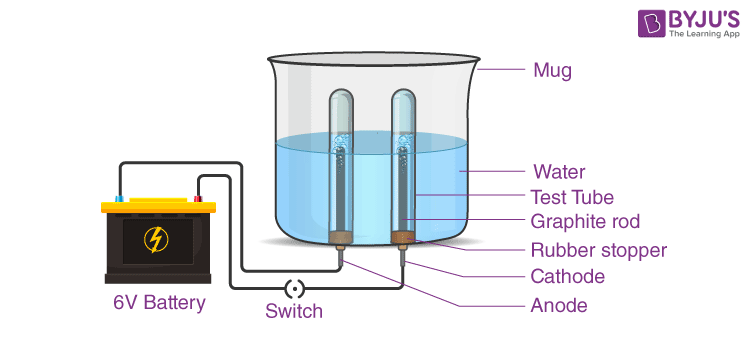1. Comment on the experiment.
2. Identify the gases that evolved.
3. Give a chemical test for distinguishing the two gases.
4. Write the chemical reactions taking place on anode and cathode.
5. Is the volume of the gas collected the same in both the test tubes?

Q-5: What is a double decomposition reaction?

Click the PDF to check the answers for Practice Questions.

## Recommended Videos